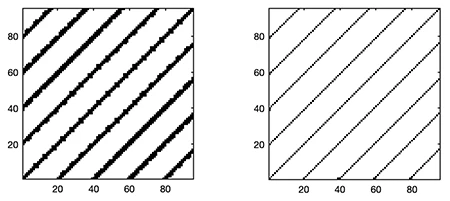# CRP Toolbox

## skel_crp

Removes thickening of diagonal lines in RP.

### Syntax

``````y=skel_crp(x);
``````

### Description

y = skel_crp(x) creates a new recurrence matrix y in which all recurrence points of the recurrence matrix x are removed which lead to a thickening of diagonal lines. Slubs, but also block structures are removed in favour of the longest diagonal lines (skeletonization). Whenever a diagonal line (starting with the longest lines contained in the diagonal line length histogram) encounters an adjacent diagonal line, this adjacent line and – recursively – all its consecutive adjacent lines, get deleted.

### Examples

``````a = sin(linspace(0,5*2*pi,100));
X = crp(a,2,5,.5,'nonorm','nogui');
Y = skel_crp(X);
nexttile
imagesc(X)
axis xy square
nexttile
imagesc(Y)
axis xy square
colormap([1 1 1; 0 0 0])
``````